Design counter for given sequence

• Difficulty Level : Medium
• Last Updated : 11 Dec, 2020

Prerequisite – Counters
Problem – Design synchronous counter for sequence: 0 → 1 → 3 → 4 → 5 → 7 → 0, using T flip-flop.

Explanation – For given sequence, state transition diagram as following below: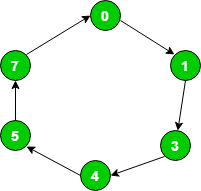State transition table logic:

Present StateNext State
01
13
34
45
57
70

State transition table for given sequence:

Present StateNext State
Q3Q2Q1Q3(t+1)Q2(t+1)Q1(t+1)
000001
001011
011100
100101
101111
111000

T flip-flop – If value of Q changes either from 0 to 1 or from 1 to 0 then input for T flip-flop is 1 else input value is 0.

QtQt+1T
000
011
101
110

Draw input table of all T flip-flops by using the excitation table of T flip-flop. As nature of T flip-flop is toggle in nature. Here, Q3 as Most significant bit and Q1 as least significant bit.

Input table of Flip-Flops
T3T2T1
001
010
111
001
010
111

Find value of T3, T2, T1 in terms of Q3, Q2, Q1 using K-Map (Karnaugh Map):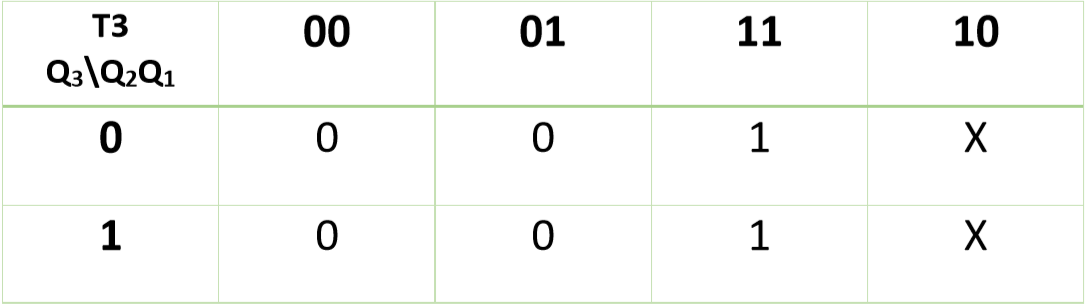Therefore,

T3 = Q2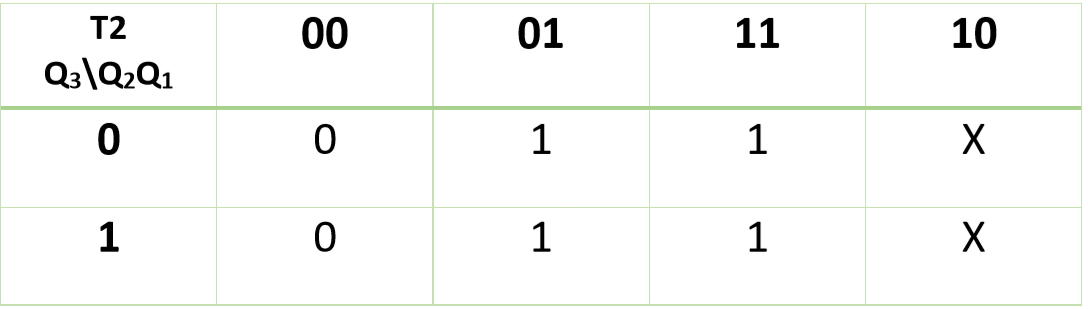Therefore,

T2 = Q1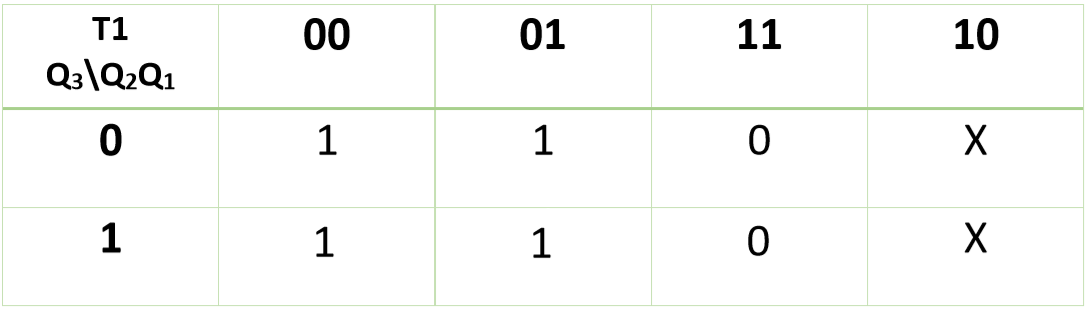Therefore,

T1 = Q2

Now, you can design required circuit using expressions of K-maps: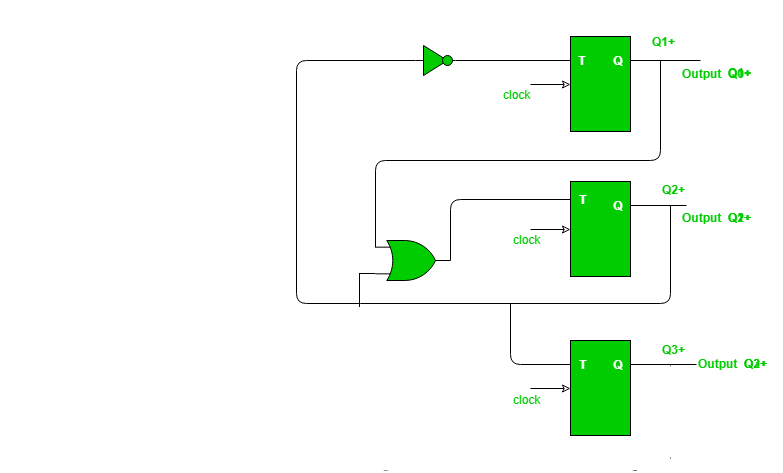My Personal Notes arrow_drop_up